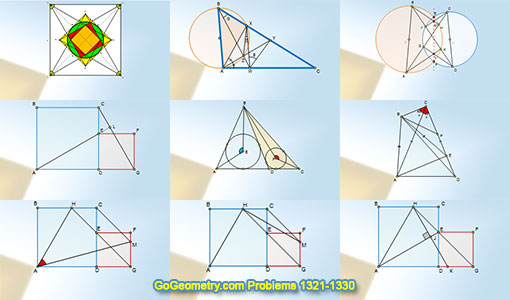# Geometry Problems 1321-1330. Level: High School, CollegeGeometry Problems 1321 - 1330Geometry Problem 1321. Intersecting Circles, Diameter, Secant, Chord, Collinearity. Geometry Problem 1322. Triangle, Angle Bisector, Circumcircle, Chord, Secant, Sum of two Angles. Geometry Problem 1323. Square, Angle Trisector, Diagonal, Regular Dodecagon, Area, 30-60 degrees. Geometry Problem 1324. Quadrilateral, Diagonal, 45 Degrees, Angle Bisector, Isosceles Triangle, Congruence. Geometry Problem 1325. Octagons, Midpoints, Squares, Mobile Apps, iPad. Geometry Problem 1326. Triangle, Cevian, Incenters, Sum of Angles, 270 Degrees. Geometry Problem 1327. Two Squares Side by Side, Perpendicular, 90 Degrees, Angle. Geometry Problem 1328. Two Squares Side by Side, Perpendicular, 90 Degrees, Congruence. Geometry Problem 1329. Two Squares Side by Side, Parallel, Perpendicular, 90 Degrees, Congruence. Geometry Problem 1330. Two Squares Side by Side, Parallel, 45, 90 Degrees.

Home | Sitemap | Geometry | Problems | Visual Index | 10 ProblemsEmail | Post a comment | by Antonio Gutierrez
Last updated: Mar 8, 2018